×

Search anything:

# Bidirectional Search

#### Algorithms Graph Algorithms Problems on Binary TreeGet this book -> Problems on Array: For Interviews and Competitive Programming

Bidirectional Search is Graph Search Algorithm where two graph traversals (BFS) take place at the same time and is used to find the shortest distance between a fixed start vertex and end vertex. It is a faster approach, reduces the time required for traversing the graph. It can be used for other applications as well.

It significantly reduces the amount of exploration done. It is implemented using the Breadth First Search (BFS) Algorithm. (If you don't know what BFS is refer to this article first). BFS is run simultaneously on two vertices - the start and the end vertex. One single BFS tree is now replaced by two sub trees, and the search is terminated when the two trees intersect.

## Working

Let's try to understand the working of Bidirectional search algorithm through the following example.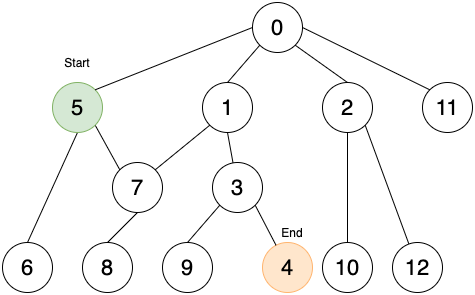The start node is 5 and the end node is 4.
Aim: To find the shortest path from 5 to 4 using bidirectional search.

Do BFS from both directions.

1. Start moving forward from start node (Green) and backwards from end node (Orange).2. Similar to BFS, at every point explore the next level of nodes till you find an intersecting node.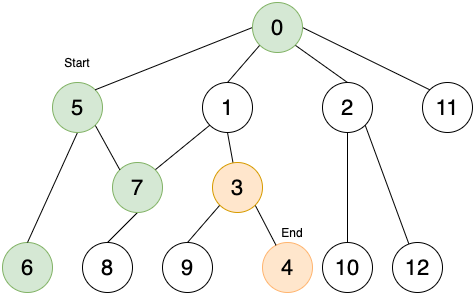3. Stop on finding the intersecting node.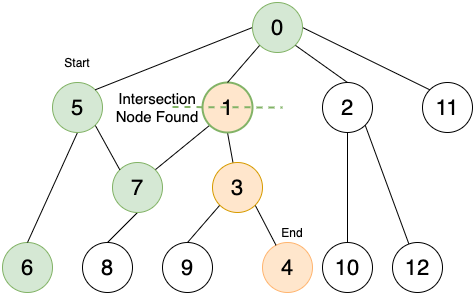4. Trace back to find the path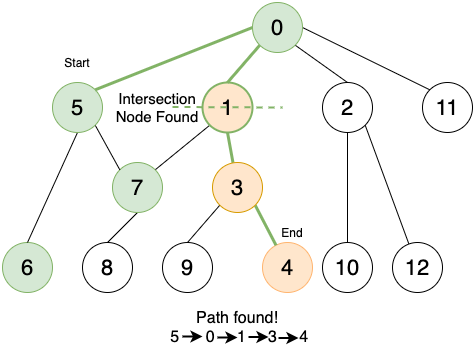## Pseudocode

``````startq = Queue for BFS from start node
endq = Queue for BFS from end node
parent= Array where startparent[i] is parent of node i
visited= Array where visited[i]=True if node i has been encountered
while startq is not empty and endq is not empty
perform next iteration of BFS for startq (also save the parent of children in parent array)
perform next iteration of BFS for endq
if we have encountered the intersection node
save the intersection node
break
using intersection node, find the path using parent array
``````

## Bidirectional search vs BFS

Let solve the the same example using BFS. Find the shortest path from node 5 to node 4 using BFS,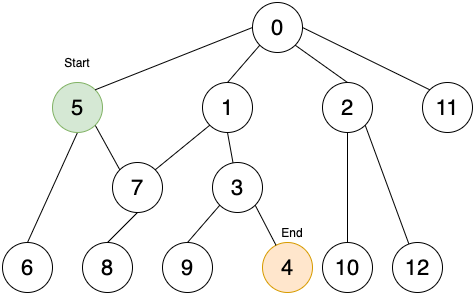Iteration 1.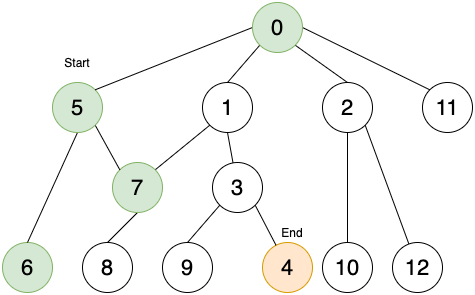Iteration 2.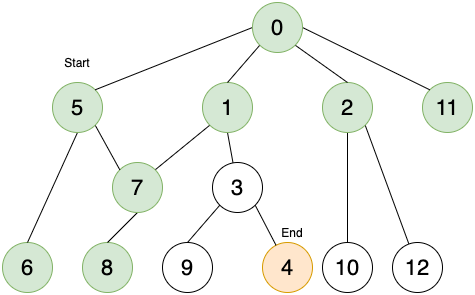Iteration 3.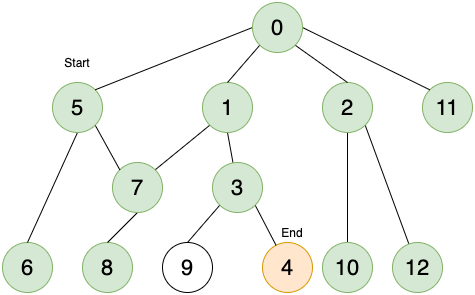Finally, we reach node 4.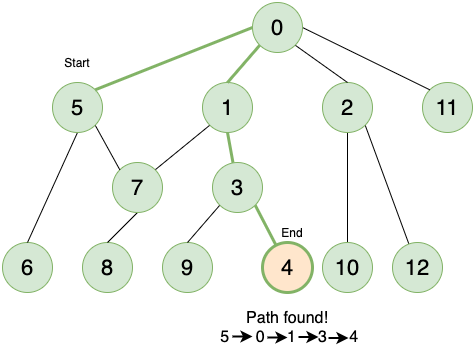Comparing results from BFS and Bidirectional Search: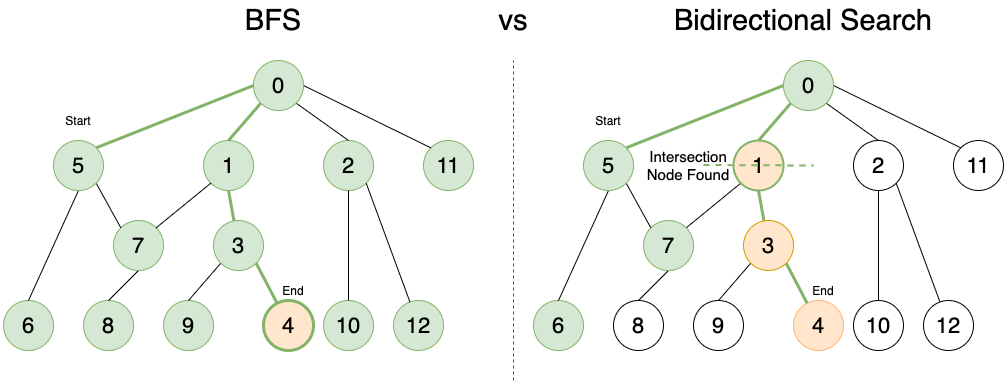We see that Bidirectional search not only required less iterations but we also visited much lesser nodes. This would prove to be very useful when the size of the graph is very large and the cost of travelling in both directions is the same. Hence, bidirectional search is a more efficient algorithm.

## Implementation

``````// Part of OpenGenus
import java.util.*;

class Graph{
int V; // Number of veritces
int E; // Number of edges
Graph(int v, int e){
V=v;
E=e;
for(int i=0;i<V;i++){
}
}
}
void BFS(ArrayList<Integer> queue, boolean[] visited, int[] parent){
int current=queue.remove(0);
if(!visited[x]){
visited[x]=true;
parent[x]=current;
}
}
}
}
class BidirectionalSearch{
static String BidirectionalSearchPath(Graph G, int src, int dest){
String path="";
boolean[] Visited1=new boolean[G.V];
boolean[] Visited2=new boolean[G.V];
int[] parent1=new int[G.V];
int[] parent2=new int[G.V];
for(int i=0;i<G.V;i++){
Visited1[i]=false;
Visited2[i]=false;
parent1[i]=-1;
parent2[i]=-1;
}
ArrayList<Integer> queue1=new ArrayList<>();
ArrayList<Integer> queue2=new ArrayList<>();
Visited1[src]=true;
Visited2[dest]=true;
int intersection=-1;
while(queue1.size()>0 && queue2.size()>0 && intersection==-1){
G.BFS(queue1, Visited1, parent1);
G.BFS(queue2, Visited2, parent2);

for(int i=0;i<G.V;i++){
if(Visited1[i]&& Visited2[i]){  //checking intersection
intersection=i;
break;
}
}
}

if(intersection==-1)
return "";

String path1="";
int j=intersection;
while(j!=-1){
path1=j+" "+path1;
j=parent1[j];
}
String path2="";
j=parent2[intersection];
while(j!=-1){
path2=path2+j+" ";
j=parent2[j];
}
path=path1+path2;
return path;
}

public static void main(String[] args) {
Scanner inp=new Scanner(System.in);
System.out.println("Enter the number of vertices");
int v=inp.nextInt();
System.out.println("Enter the number of edges");
int e=inp.nextInt();
Graph G=new Graph(v, e);
System.out.println("Edges:");
for(int i=0;i<G.E;i++){
int x=inp.nextInt();
int y=inp.nextInt();
}
String path=BidirectionalSearchPath(G, 0, 2);
if(path.equals(""))
System.out.println("Path does not exist");
else
System.out.println("Path : " + path);
}
}
``````

# Complexity

If b is the branching factor(the maximum number of successors of any node) of the tree, and distance between the start and end vertex is d, normal BFS/DFS complexity is O(b^d). In the case of Bidirectional Search, we run two simultaneous search operations with the complexity of each operation as O(b^(d/2)) which makes the total complexity as O(b^(d/2)+b^(d/2)). This clearly is significantly less than O(b^d).

For a Binary tree, branching factor b is 2 and the depth of tree d is of the order O(log N) for a balanced tree with N elements so:

• Time complexity is O(N) for balanced tree

The time complexity is same as that of Breadth First search or Depth First Search but in practice, Bidirectional search performs significantly better.

## Question

#### Which of the following algorithms is used as a part of the Bidirectional Search Algorithm?

Depth First Search
both (a) and (b)
neither (a) or (b)
Bidirectional Search uses Breadth First Search simultaneously from the start and end vertex.

With this article at OpenGenus, you must have the complete idea of this powerful technique of Bidirectional search. Enjoy.#### Sargam Monga

Intern at OpenGenus | Student at Indraprastha Institute of Information Technology, New Delhi

Bidirectional Search